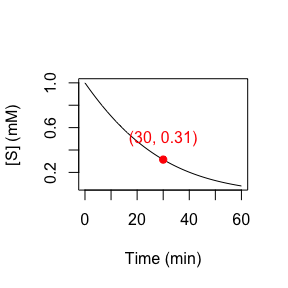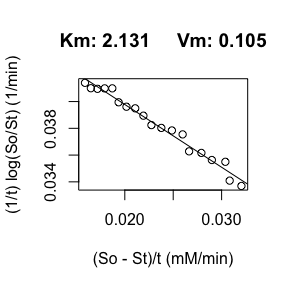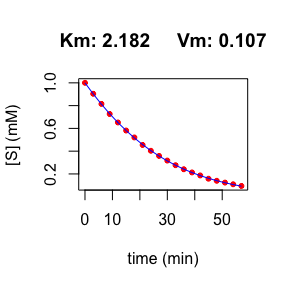# Michaelis-Menten and the Lambert W function

library(renz)

## Stating the problem we want to solve

For the sake of concretion, let’s consider a michaelian enzyme with $$Km = 2$$ mM and $$V_{max} = 0.1$$ mM/min, which catalyzes an essentially irreversible reaction (the rate of the inverse reaction is negligible). Now, we start the reaction a time 0 with a substrate concentration of 1 mM. Can we predict the time-course of the substrate concentration? For instance, what would be the concentration of substrate remaining after 30 min?

Of course we can! The function sE.progress() can do this job for us:

d <- sE.progress(So = 1, time = 60, Km = 2, Vm = 0.1, plot = FALSE)

We can now plot the results:

plot(d$t, d$St, ty = 'l', xlab = "Time (min)", ylab = "[S] (mM)")
points(d$t[which(d$t == 30)], d$St[which(d$t == 30)], pch = 19, col = "red")
text(30, 0.5, paste("(30, ", round(d$St[which(d$t == 30)], 2), ")", sep = ""), col = "red")However, the theoretical principles that allow us to find the desired solution are not so trivial. The function sE.progress(), that we have used to predict [S] after 30 min of reaction, as well as the function fE.progress that estimates the kinetic parameters given a single progress-curve for the substrate, both invoke the so-called Lambert W function. For the reader interested in the theory behind the scene, we will provide next some orientation.

## The integrated Michaelis-Menten

Given that the Michaelis-Menten equation is the derivative of substrate concentration with respect to time, its integral should inform us of how the substrate concentration varies over time, which is our aim. Thus, let’s integrate it.

$\begin{equation} \tag{1} v = \frac{-d[S]}{dt} = V_{max} \frac{[S]}{[S] + K_m} \end{equation}$

$\begin{equation} \tag{2} \int_{S_o}^{S_t} \frac{[S] + K_m}{[S]} d[S] = -\int_{0}^t V_{max} dt \end{equation}$

$\begin{equation} \tag{3} \frac{1}{t} \ln \frac{S_o}{S_t} = - \frac{1}{K_m} \frac{(S_o - S_t)}{t} + \frac{V_{max}}{K_m} \end{equation}$

We can easily rewrite this last equation in exponential form:

$\begin{equation} \tag{4} \frac{S_t}{K_m} e^{\frac{S_t}{K_m}} = \frac{S_o}{K_m} e^{\frac{-V_{max}t + S_o}{K_m}} \end{equation}$

At this point we face a problem: how to solve the variable $$S_t$$? This where the Lambert W function comes into play. So, before showing the solution, let’s introduce this useful function.

## The Lambert W function

Let’s consider the function $$y = x e^x$$. Given a value of $$x$$, it is straightforward to compute the $$y$$-value. However, we will be interested in the inverse operation, that is, given a value of $$y$$, find the corresponding value of $$x$$. Thus, we define the Lambert W function as:

$\begin{equation} \tag{5} W(y) = x \end{equation}$

To find the desired value of $$x$$ we can define the function $$f(x) = xe^x -y$$ and resort to numerical methods to find their roots. To this end, we can use the Newton method:

$\begin{equation} \tag{6} x_{n+1} = x_n - \frac{f(x_n)}{f'(x_n)} \end{equation}$

Let’s see how to proceed with a concrete example. Let’s say we want to know what value of $$x$$ satisfies the equation $$1 = xe^x$$. Then, the function for which we have to search their roots will be $$f(x) = xe^x - 1$$. Thus, using the Newton method, we will have to iterate for

$\begin{equation} \tag{7} x_{n+1} = x_n - \frac{xe^x - 1}{e^x(1 + x)} \end{equation}$

Next, we show the first 5 iterations, starting with a seed x = 0:

x <- 0
for(i in 1:5){
x <- x - (x*exp(x) -1)/(exp(x)*(1 + x))
print(paste("iteration ", i, ":    x = ", x, ", y = ", x*exp(x), sep = ""))
}
#>  "iteration 1:    x = 1, y = 2.71828182845905"
#>  "iteration 2:    x = 0.683939720585721, y = 1.35534255099475"
#>  "iteration 3:    x = 0.57745447715445, y = 1.02873388682923"
#>  "iteration 4:    x = 0.567229737730117, y = 1.00023889012357"
#>  "iteration 5:    x = 0.567143296530296, y = 1.00000001691234"

Just for the sake of curiosity, $$W(1) = \Omega$$. That is, the value of $$x$$ we have found, 0.567…, is named omega.

## Fitting the progress curve for enzyme-catalyzed reactions

Now that we know how to solve the $$S_t$$ variable from equation (4) using the approach allowed by the Lambert W function (in the Enzymology field this is known as the Schnell-Mendoza equation), we will illustrate how the function fE.progress, which makes use of the Schnell-Mendoza equation, allows us to obtain, from one single progress curve, the kinetic parameters of the enzyme.

Suppose we have obtained the following data for from a progression curve:

t S
0 1.0000000
3 0.9038617
6 0.8150139
9 0.7265242
12 0.6520818
15 0.5813286
18 0.5204660
21 0.4545402
24 0.4032604
27 0.3581450
30 0.3175182
33 0.2765952
36 0.2412796
39 0.2133202
42 0.1867971
45 0.1581128
48 0.1398343
51 0.1238722
54 0.1092823
57 0.0944481

If we use these data to build a dataframe call “data”, then fE.progress(data) will fit the progression curve to estimate $$K_m$$ and $$V_{max}$$:

fE.progress(data)#> 8.612207e-05 (2.13e-01): par = (2.131 0.105)
#> 8.239456e-05 (4.17e-03): par = (2.181273 0.1068345)
#> 8.239313e-05 (5.03e-06): par = (2.182215 0.1068686)#> $parameters #> Km Vm #> 2.182 0.107 #> #>$data
#>     t         St  fitted_St
#> 1   0 1.00000000 1.00000000
#> 2   3 0.90386170 0.90260064
#> 3   6 0.81501389 0.81211182
#> 4   9 0.72652424 0.72842166
#> 5  12 0.65208176 0.65137096
#> 6  15 0.58132855 0.58075525
#> 7  18 0.52046598 0.51632848
#> 8  21 0.45454019 0.45780807
#> 9  24 0.40326039 0.40488110
#> 10 27 0.35814496 0.35721132
#> 11 30 0.31751821 0.31444643
#> 12 33 0.27659516 0.27622548
#> 13 36 0.24127961 0.24218575
#> 14 39 0.21332023 0.21196908
#> 15 42 0.18679715 0.18522723
#> 16 45 0.15811275 0.16162628
#> 17 48 0.13983433 0.14085003
#> 18 51 0.12387221 0.12260230
#> 19 54 0.10928234 0.10660847
#> 20 57 0.09444807 0.09261609

Note that for comparison purposes, this function also fits the linearized integrated equation